### Home > CALC > Chapter 8 > Lesson 8.3.2 > Problem8-114

8-114.
1. Integrate the following problems without using your calculator. Be sure to change your bounds if you use u-substitution. Homework Help ✎

1.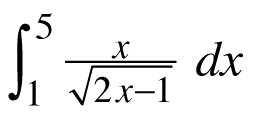2.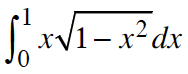3.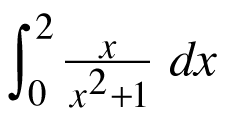4.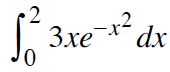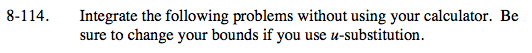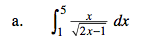$\text{Then }x=\frac{1}{2}(U+1).$

Let U = 2x − 1.

$\frac{dU}{dx}=2$

$\frac{1}{2}dU=dx$

Rewrite the integrand and don't forget to write the bounds in terms of U.

$=\int_1^9\frac{U+1}{4\sqrt{U}}dU=\frac{1}{4}\int_1^9(U^{1/2}+U^{-1/2})=\frac{16}{3}$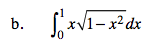Let U = 1 − x²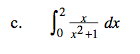$=\int_1^5\frac{1}{2u}du=\frac{1}{2}\ln(u)\Big|_1^5=\frac{1}{2}\ln(5)$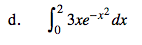$=\int_0^{-4}-\frac{3}{2}e^udu=\frac{3}{2}-\frac{3}{2}e^{-4}$